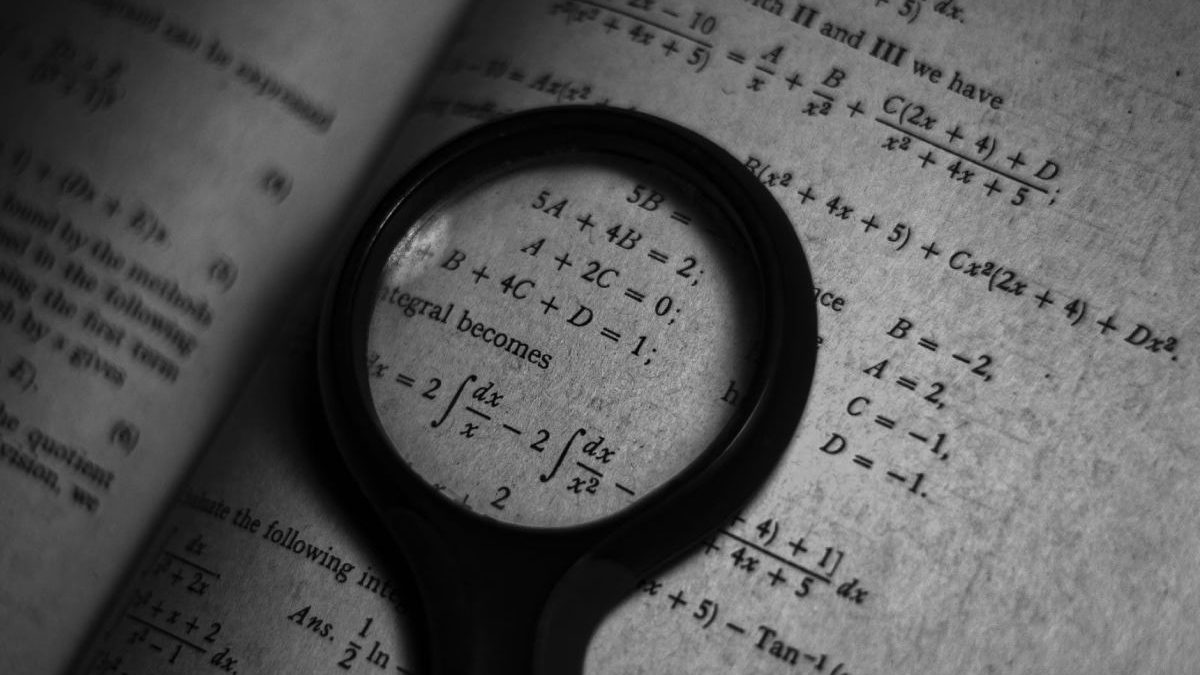08 Dec 2023

## Blog Post# How to Learn the Fibonacci Series with Applications?

Have you ever asked yourself this question, why are you studying math? If not, this is the question to ask yourself. In fact, this is a question you should always ask yourself before you start learning, as it sets the goal and agenda for learning. Once you know the answer you will successfully learn that thing. Furthermore,  there are three main aspects of learning mathematics that are calculation, application, and inspiration. Mathematics is a subject that includes logic, creative and critical thinking. Fibonacci series is the simplest series and anyone can understand it quickly if they concentrate properly.

As you know first we should understand the numbers hence in school they teach us numbers and basic operations such as addition, subtraction, and many more. Then later as you go in high school you meet two new parts of mathematics that are algebra and geometry. No matter in which field you make your career after school whether it is science, commerce, or arts you need to know both parts of mathematics in the school. Then only you can use them later in your career. And if you are someone who wishes to make their career in mathematics then they should focus on Algebra and Geometry. Especially if you want to take higher studies in the calculus section then you should be thorough with every Algebra topic from line equations to solving matrices. If you wish to learn programming languages then you should definitely understand the Fibonacci series. Let’s see some facts about this series:

• You must have seen examples of this series where you have to add the preceding number and the next number. That is one plus one is two, and then one plus two is three, then one plus two is three, then two plus three is five and the sequence goes on.
• The fun fact about this series is it appears in nature. The most interesting examples where we observe these series are flowers of petals, spirals of sunflowers and pineapple, and many more.
• If you understand these series then you will understand the several patterns of this series. If you write the squares of this series then you will see this pattern if you add those squares just like you add in this series one plus one-two, one plus four is five, four-plus nine is thirteen and the sequence goes on.
• When you do the above procedure where you add squares of this series in the same format you get the sequence of this series in odd positions, that is it does not give us the numbers that are there in the even positions in this series.
• You can explain to your kid the concept behind this series through a simple example, draw a square of one by one, near that draw one more such square, beneath that draw two by two square, next to that draw three by three square, below that draw five by five square and next to that draw eight by eight square.
• Now here you will observe a big rectangle, if you are asked to find out the area of this rectangle then you can find it in two ways. The first way is simply adding the areas of squares present inside that rectangle.
• The second way is by using the formula for the area of a rectangle that is the multiplication of length and width. So in this rectangle, the width is eight, and the length five plus eight is thirteen which is the next number in this series after eight.
• Now you can observe in both ways the final term is eight into thirteen and hence this proves this series from an application point of view.

By now, you should understand how easy math is when you study it through real-world examples and solutions. Moreover, how you can solve problems based on the Fibonacci sequence. You can understand math more practically if you learn it from Cuemath. Here you will know all the properties of each shape through practical examples. Make mathematics interesting for your children. Don’t let your child think that math is the most boring and complicated subject. Teach them conceptual math so they can enjoy math.